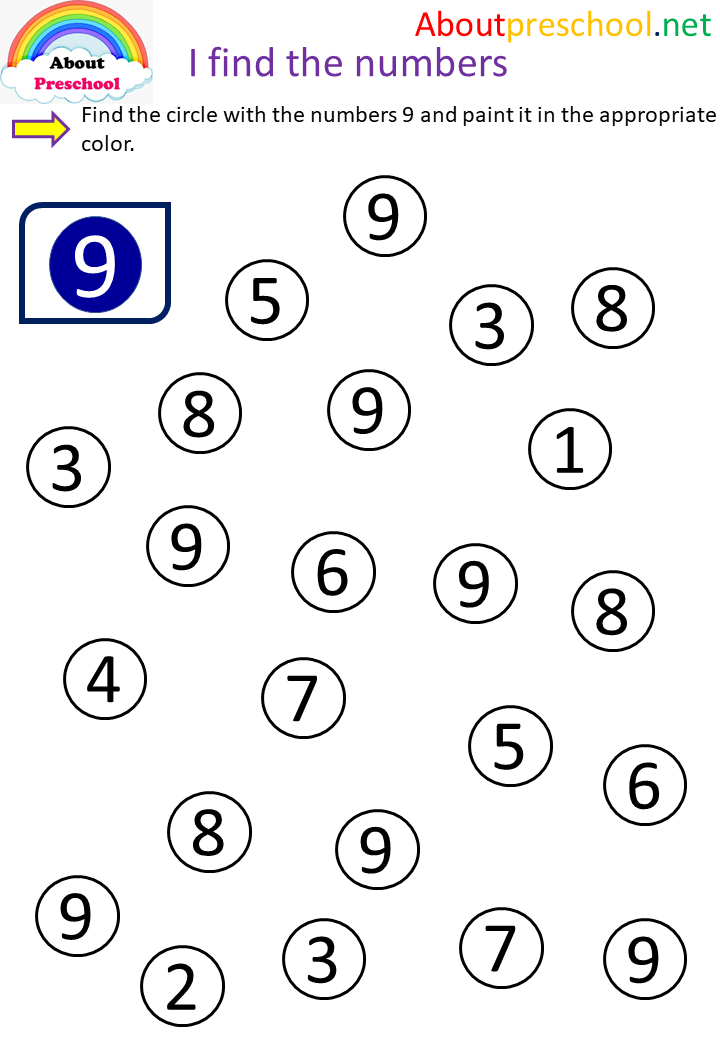I find the numbers 9 - About Preschool

# I find the numbers 9Studies on the Concept of Number

• Finding the match of the number drawn as a model among numbers from 1 to 10 mixedly arranged
• Finding the spoken number among numbers from 1 to 10 in a mixed order
• Finding the number shown among the numbers from 1 to 10 in a mixed order
• Asking the child to count from 1 to 10 with verbal directions
• Counting between given numbers (such as 5-9)
• Counting down from the given number
Match different numbers of objects
• Saying which of the two written numbers (such as 5 and 9) is less or more
• Line up a set of numbers, read and ask to show the desired number
• Count two given groups of objects and determine if they match the given number
• Count two given groups of objects and tell which one is less or more
• Saying which number is greater than given by a group of objects
• Requesting matching by giving object groups and numbers
• Saying the least (most) of the three object groups by counting the objects
• Giving a number, asking him to show the appropriate number of object groups to that number.